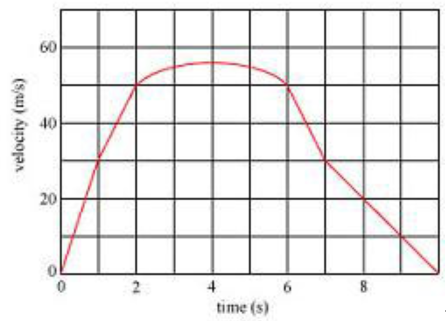# Problem: A. Find the maximum velocity Vmax of the car during the ten-second interval depicted in the graph. B. Find the maximum acceleration a max of the carC. Find the minimum magnitude of the acceleration amin of the car

###### FREE Expert Solution

A.

The maximum velocity is the highest function, where the function can be taken on.

Referring to the velocity-time graph, the maximum value of the velocity is the highest point of the graph, which is 56 m/s.

99% (250 ratings)###### Problem DetailsA. Find the maximum velocity Vmax of the car during the ten-second interval depicted in the graph.

B. Find the maximum acceleration a max of the car

C. Find the minimum magnitude of the acceleration amin of the car